Related Articles

# expr command in Linux with examples

• Difficulty Level : Medium
• Last Updated : 15 May, 2019

The expr command in Unix evaluates a given expression and displays its corresponding output. It is used for:

• Basic operations like addition, subtraction, multiplication, division, and modulus on integers.
• Evaluating regular expressions, string operations like substring, length of strings etc.

Syntax:

```\$expr expression
```

Options:

Below are some examples to demonstrate the use of “expr” command:

1. Using expr for basic arithmetic operations :

```\$expr 12 + 8
```

Example: Multiplication

```\$expr 12 \* 2
```

Output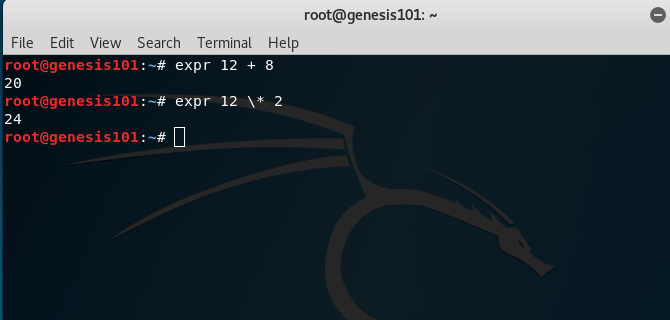Note:The multiplication operator * must be escaped when used in an arithmetic expression with expr.

2. Performing operations on variables inside a shell script

Example: Adding two numbers in a script

```echo "Enter two numbers"

sum=`expr \$x + \$y`

echo "Sum = \$sum"
```

Output: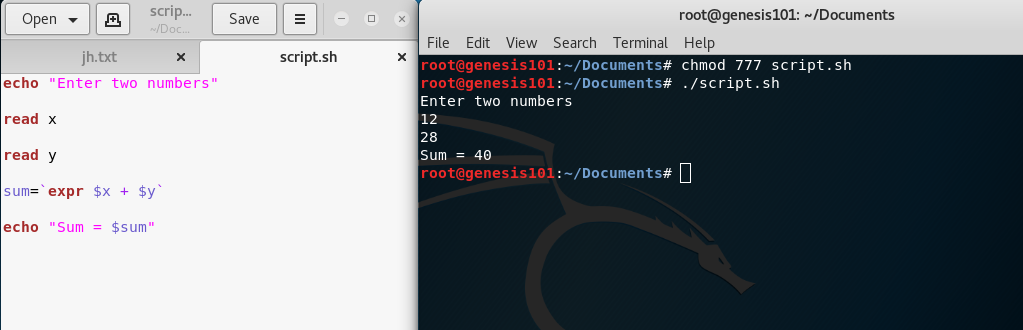Note: expr is an external program used by Bourne shell. It uses expr external program with the help of backtick. The backtick(`) is actually called command substitution.

3. Comparing two expressions

Example:

```x=10

y=20

# matching numbers with '='
res=`expr \$x = \$y`
echo \$res

# displays 1 when arg1 is less than arg2
res=`expr \$x \< \$y`
echo \$res

# display 1 when arg1 is not equal to arg2
res=`expr \$x \!= \$y`
echo \$res
```

Output: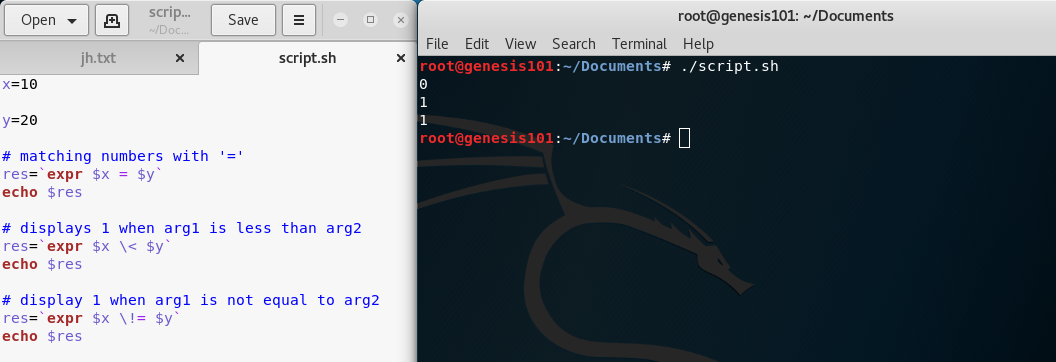Example: Evaluating boolean expressions

```# OR operation
\$expr length  "geekss"  "<"  5  "|"  19  -  6  ">"  10
```

Output: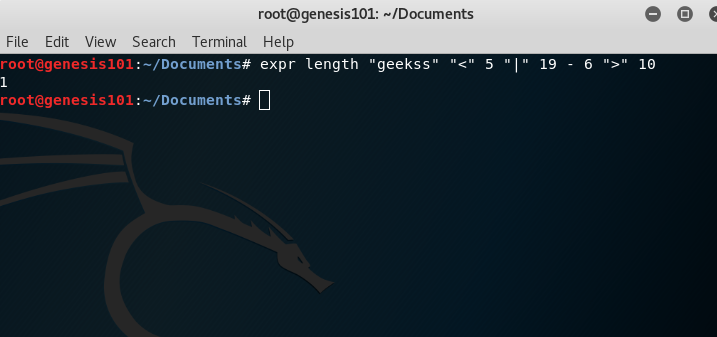```# AND operation
\$expr length  "geekss"  "<"  5  "&"  19  -  6  ">"  10
```

Output: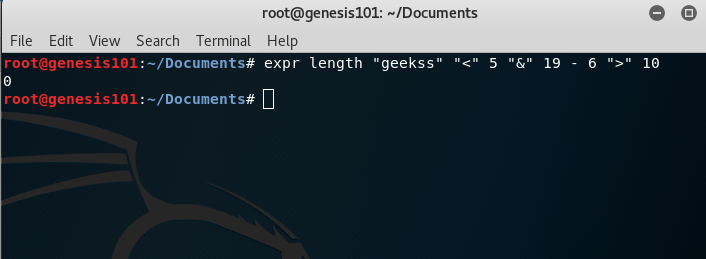4. For String operations

Example: Finding length of a string

```x=geeks

len=`expr length \$x`

echo \$len
```

Output: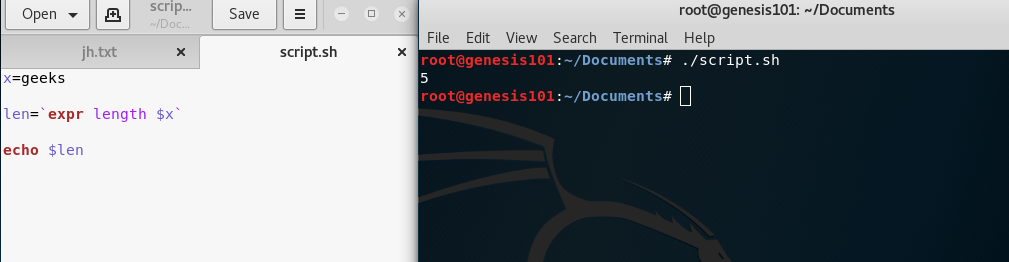Example: Finding substring of a string

```x=geeks

sub=`expr substr \$x 2 3`
#extract 3 characters starting from index 2

echo \$sub
```

Output: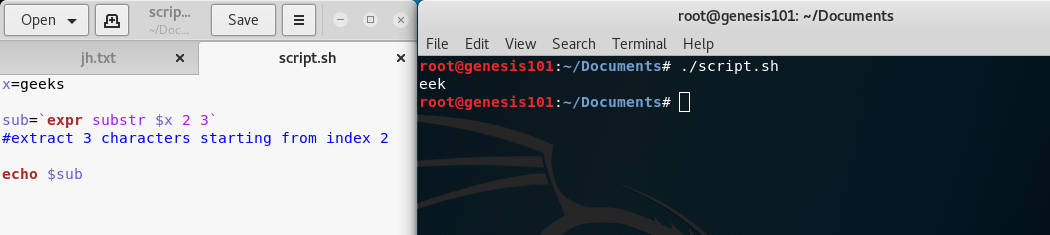Example: Matching number of characters in two strings

```\$ expr geeks : geek
```

Output: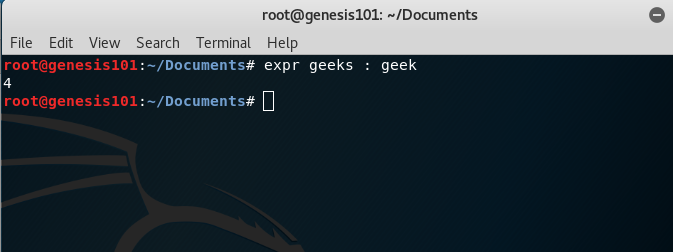My Personal Notes arrow_drop_up